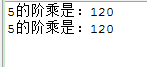# JAVA基础再回首（二十一）——递归、IO流概述、字节流写数据、读取数据、复制数据、字节缓冲流

## 递归

• 递归
• 方法定义中调用方法本身的现象

• 递归解决问题的思想
• 做递归要写一个方法
• 找到出口条件
• 找到规律

• 递归一定要有出口，否则就是死递归
• 递归的次数不能太多，否则就内存溢出
• 构造方法不能递归使用

//首先我们知道要实现5的阶乘，有两种方案
//一种是循环实现，一种是递归实现。我们这两种方案都实现一下
public class DiGuiDemo {
public static void main(String[] args) {
int jc = 1;
for (int x = 2; x <= 5; x++) {
jc *= x;
}
System.out.println("5的阶乘是：" + jc);

System.out.println("5的阶乘是："+jieCheng(5));
}
/*
* 做递归要写一个方法:
*      返回值类型：int
*      参数列表：int n
* 出口条件:
*      if(n == 1) {return 1;}
* 规律:
*      if(n != 1) {return n*方法名(n-1);}
*/
public static int jieCheng(int n){
if(n==1){
return 1;
}else {
return n*jieCheng(n-1);
}
}
}## IO流概述

• IO流概述
• IO流用来处理设备之间的数据传输
• 上传文件和下载文件
• Java对数据的操作是通过流的方式
• Java用于操作流的对象都在IO包中
• IO流分类
• 按照数据流向
• 输入流 读取数据
• 输出流 写出数据
• 按照数据类型
• 字节流
• 字节输入流 读取数据 InputStream
• 字节输出流 写出数据 OutputStream
• 字符流
• 字符输入流 读取数据 Reader
• 字符输出流 写出数据 Writer

• 如果数据所在的文件通过windows自带的记事本打开并能读懂里面的内容，就用字符流。其他用字节流。
• 如果你什么都不知道，就用字节流

• IO流常用基类（这个我们上面也提到了）
• 字节流的抽象基类：
• InputStream ，OutputStream。
• 字符流的抽象基类：
• Reader ， Writer。

• 如：InputStream的子类FileInputStream。

## 字节流写数据

OutputStream它的子类FileOutputStream

• FileOutputStream的构造方法
• FileOutputStream(File file)
• FileOutputStream(String name)
public class FileOutputStreamDemo {
public static void main(String[] args) throws IOException {
// 创建字节输出流对象（FileOutputStream的这两种构造方法效果是一样的用那个都可以）

// FileOutputStream(File file)
// File file = new File("fos.txt");
// FileOutputStream fos = new FileOutputStream(file);

// FileOutputStream(String name)
FileOutputStream fos = new FileOutputStream("fos.txt");

//写数据
fos.write("helloworld".getBytes());
fos.write("java".getBytes());

//释放资源
//关闭此文件输出流并释放与此流有关的所有系统资源。
fos.close();
}
}

• 我们最后为什么一定要close()呢?

• 让流对象变成垃圾，这样就可以被垃圾回收器回收了
• 通知系统去释放跟该文件相关的资源
• 字节输出流操作步骤：

• A：创建字节输出流对象
• B：写数据
• C：释放资源

这总结写的么问题吧，让你的思路更清晰，代码写的更流畅

## 字节流写数据的方式

• public void write(int b) ：写一个字节
• public void write(byte[] b)：写一个字节数组
• public void write(byte[] b,int off,int len)：写一个字节数组的一部分

        // 调用write()方法
fos.write(97); //输出结果是97 -- 底层二进制数据 -- 通过记事本打开 -- 找97对应的字符值 -- a

//public void write(byte[] b):写一个字节数组
byte[] bys={97,98,99,100,101};
fos.write(bys);//输出结果是abcde

//public void write(byte[] b,int off,int len):写一个字节数组的一部分
fos.write(bys,1,3);//输出结果是bcd

## 字节流读取数据

InputStream的子类FileInputStream

• FileInputStream的构造方法
• FileInputStream(File file)
• FileInputStream(String name)
• FileInputStream的成员方法
• public int read()：一次读取一个字节
• public int read(byte[] b)：一次读取一个字节数组

• A：创建字节输入流对象
• C：释放资源

    //我们在项目中新建一个fis.txt文件，并写入helloword数据，然后用代码实现读取它
public class FileInputStreamDemo {
public static void main(String[] args) throws IOException {
//创建字节输入流对象
FileInputStream fis = new FileInputStream("fis.txt");

int by = 0;
// 读取，赋值，判断
while ((by = fis.read()) != -1) {
System.out.print((char) by);
}

// 释放资源
fis.close();
}
}

    // 数组的长度一般是1024或者1024的整数倍
byte[] bys = new byte;
int len = 0;
while ((len = fis.read(bys)) != -1) {
System.out.print(new String(bys, 0, len));
}

## 字节流复制数据

• 数据源：从哪里来
• a.txt – 读取数据 – FileInputStream
• 目的地：到哪里去
• b.txt – 写数据 – FileOutputStream
public class CopyFileDemo {
public static void main(String[] args) throws IOException {
// 封装数据源
FileInputStream fis = new FileInputStream("a.txt");
// 封装目的地
FileOutputStream fos = new FileOutputStream("b.txt");

int by = 0;
while ((by = fis.read()) != -1) {
fos.write(by);
}
// 释放资源(先关谁都行)
fos.close();
fis.close();
}
}

public class CopyFileDemo {
public static void main(String[] args) throws IOException {
// 封装数据源
FileInputStream fis = new FileInputStream("a.txt");
FileOutputStream fos = new FileOutputStream("b.txt");

// 复制数据
byte[] bys = new byte;
int len = 0;
while ((len = fis.read(bys)) != -1) {
fos.write(bys, 0, len);
}

// 释放资源
fos.close();
fis.close();
}
}

## 字节缓冲流

• 字节缓冲输出流
• BufferedOutputStream
• 字节缓冲输入流
• BufferedInputStream

public class BufferedOutputStreamDemo {
public static void main(String[] args) throws IOException {
// BufferedOutputStream(OutputStream out)
// 简单的写法
BufferedOutputStream bos = new BufferedOutputStream(
new FileOutputStream("bos.txt"));

// 写数据
bos.write("hello".getBytes());

// 释放资源
bos.close();
}
}

public class BufferedInputStreamDemo {
public static void main(String[] args) throws IOException {
// BufferedInputStream(InputStream in)
BufferedInputStream bis = new BufferedInputStream(new FileInputStream(
"bos.txt"));

// 读取数据
int by = 0;
while ((by = bis.read()) != -1) {
System.out.print((char) by);
}

// 释放资源
bis.close();
}
}


        byte[] bys = new byte;
int len = 0;
while ((len = bis.read(bys)) != -1) {
System.out.print(new String(bys, 0, len));
}